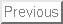Exercises to Accompany
A Guide to First-Passage Processes

This is a modest list of exercises to accompany A Guide to First-Passage Processes'' and was last updated on December 8, 2002. I am indebted to Prof. R. Dorfman for the suggestion to compile some exercises.

Chapter 1:

1. Determine the asymptotic probability distribution for a random walk in one dimension when the initial probability distribution is broad. For example,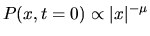, withfor.

2. Determine the asymptotic probability distribution for a one-dimensional random walk that hops 2 lattice spacings to the right with probability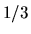or hops 1 lattice spacing to the left with probability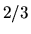. Use the techniques of section 1.3.

Chapter 2:

1. Consider a diffusing particle in the intervalthat is initially placed at. There is a drift velocity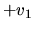for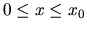and a drift velocity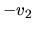for. Compute the eventual exit probabilities to each end of the interval. Compute the mean first-passage time to each end.

2. Consider a diffusing particle that is initially located at the junction of three line segments of lengths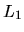,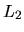, and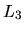, with. The particle freely diffuses in each segment, but when the end of a segment is reached the particle is absorbed. What is the probability that the particle is eventually absorbed at the end of the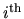segment? What is the average time for the particle to be absorbed at one of the endpoints? (That is, the identity of the absorbing segment is unspecified when absorption occurs.)  What is the average time for the particle to be absorbed at the end of thesegment? (That is, the identity of the absorbing segment is specified when absorption occurs.)

Chapter 5:

1. Consider a Cayley tree with coordination number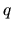that consists of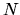generations. A random walk is initially at the root of the tree. Determine: (a) the mean first-passage time to get from the root to any site in the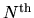generation, and (b) the mean first-passage time to get from the root to a specified site in thegeneration, with the condition that the random walk is reflected from all other sites in thegeneration.

2. Consider an alternating Cayley tree in which the number of branches at each new generation alternately bifurcates or trifurcates. Compute the probability of eventual return to the origin.

3. Compute the first-passage probability to reach one corner of an-generation Sierpinski gasket when a random walk begins at another corner of the gasket.

Chapter 6:

1. Compute the probability for a diffusing particle to eventually hit the north pole and the south pole of a unit sphere when the particle is initially on the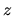-axis at a distance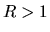from the center of the sphere. Repeat the problem for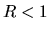.

2. Compute the probability that a diffusing particle eventually hits the northern hemisphere of a unit sphere in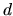dimensions when the particle is initially on the-axis at a distancefrom the center of the sphere. Repeat the problem for.

Chapter 7:

1. Consider a diffusing particle that is located at an arbitrary initial point within a rectangular domain of length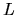and height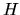. The particle is absorbed as soon as it hits the boundary. Compute the probability for the particle to hit an arbitrary point on the boundary.

2. Consider a diffusing particle that is located at one focus of an ellipse of semi-major axis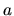and semi-minor axis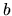. The particle is absorbed as soon as it hits the boundary. Compute the probability for the particle to hit an arbitrary point on the boundary.

Chapter 8:

1. Consider three identical diffusing particles in one dimension that are initially located at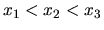. What is the mean time until the particle at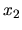hits its left neighor, its right neighbor, or either of its neighbors?